Name _____________________________Date ___________________
Properties of Parallel Lines
Complete.

 1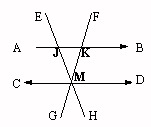Line AB is parallel to line CD.m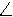KMD = 57 If mAJM = 56 and mCME = x + 108, find the value of x.
 2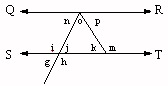Line QR is parallel to line ST.mo = 52 If mn = 52 and mk = 6x - 44, find the value of x.
 3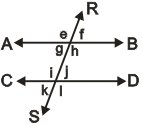Line AB is parallel to line CD. If mi = 52 and mk = 32x, find the value of x.
 4Line AB is parallel to line CD.mJMK = 45 If mEJB = 40 and mHMD = x + 118, find the value of x.
 5Line QR is parallel to line ST.mn = 57 If mp = 43 and mj = 6x - 81, find the value of x.
 6Line QR is parallel to line ST.mn = 58 If mo = 52 and mi = x + 106, find the value of x.
 7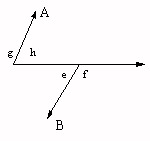Line A is parallel to line C. If mf = 85 and mh = 4x + 51, find the value of x.
 8Line A is parallel to line C. If mh = 47 and mf = 12x - 23, find the value of x.